Courses

# NABARD Manager Quant Aptitude Mock Test 3

## 20 Questions MCQ Test NABARD Manager - Mock Tests & Previous Year Papers | NABARD Manager Quant Aptitude Mock Test 3

Description
This mock test of NABARD Manager Quant Aptitude Mock Test 3 for Banking Exams helps you for every Banking Exams entrance exam. This contains 20 Multiple Choice Questions for Banking Exams NABARD Manager Quant Aptitude Mock Test 3 (mcq) to study with solutions a complete question bank. The solved questions answers in this NABARD Manager Quant Aptitude Mock Test 3 quiz give you a good mix of easy questions and tough questions. Banking Exams students definitely take this NABARD Manager Quant Aptitude Mock Test 3 exercise for a better result in the exam. You can find other NABARD Manager Quant Aptitude Mock Test 3 extra questions, long questions & short questions for Banking Exams on EduRev as well by searching above.
QUESTION: 1

### Rahul is younger than Radha by 10 yrs. If 5 yrs back their ages were in the ratio 1:2, how old is Radha?

Solution:

Let Rahul age = x years
⇒ Radha's age = (x + 10)years
⇒ x - 5 /x + 10-5 = 1/ 2
⇒ 2x - 10 = x + 5
⇒ x = 15 years
Hence, Radha's age = (15 + 10)years = 25 years

QUESTION: 2

### A circular swimming pool is surrounded by a concrete wall 4m wide. If the area of the concrete wall surrounding the pool is 11 25 that of the pool, then the radius (in m) of the pool is

Solution: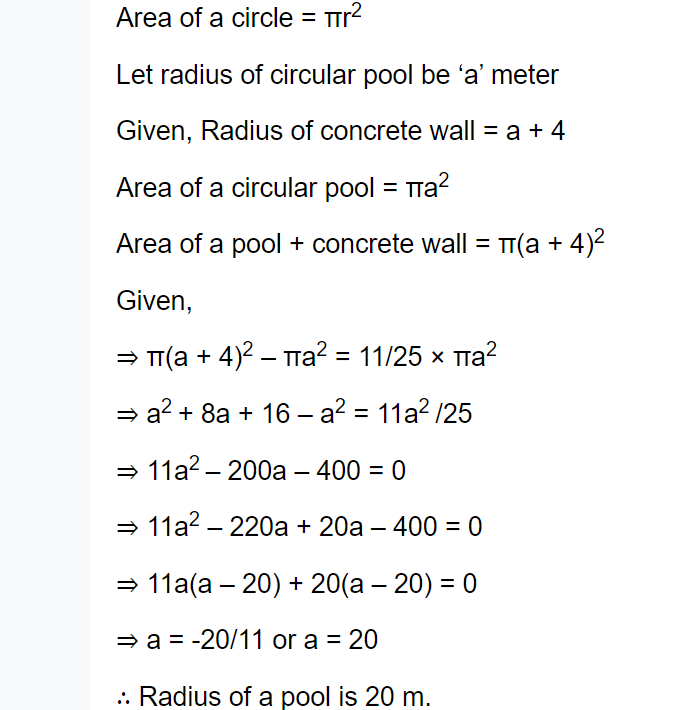QUESTION: 3

### The quantity of water in litres that must be added to 12 litres of beer priced at Rs 10 a litre to reduce the price to Rs 8 a litre is

Solution:
QUESTION: 4

Of the 3 numbers,second is twice the first and is also thrice the third. If the average of the three numbers is 44,the largest number is

Solution:

The biggest number should be 72.

First, let’s start off by defining the numbers in terms of x. If the second number (the largest number) is defined by x, the first number is 1/2x and the third number is 1/3x. This is because the first number is half of the second number while the third number is a third of the second number.

Now that we have defined the three numbers in terms of x, let’s focus on the average given in the question. To find the average, you add up all the values and divide by the number of values.

Average= (Number 1+Number 2+Number 3)/number of values.

Since there are three numbers and the average is given, we can say

44=(1/2x+x+1/3x)/3

Multiply both sides by three

132=1/2x+x+1/3x

Add up all the like terms

132=11/6x

792=11x

x=72

QUESTION: 5
One-third of two-fifth of three-seventh of a number is 10. What is 40% of that number?
Solution:
QUESTION: 6

What is the probability of getting at least one six in a single throw of three unbiased dice?

Solution: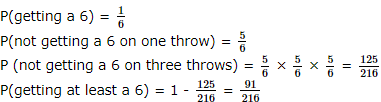QUESTION: 7
Which is the Number that comes next in the series:
8, 22, 8, 28, 8, .?
Solution: This is a simple addition series with a random number, 8, interpolated as every other number. In the series, 6 is added to each number except 8, to arrive at the next number.
QUESTION: 8

A man buys 2 dozen bananas at Rs.16 per dozen. After selling 18 bananas at the rate of Rs.12 per dozen, the shopkeeper reduced the rate to Rs.4 per dozen. The perent loss is :

Solution:

C.P. = Rs (16X 2) = 32
S.P. = Rs (12 X 1.5 + 4 X 0.5) = Rs(18 + 2) = Rs 20
∴ Loss% = ( 12 32 X 100)% = 37.5%

QUESTION: 9
The ratio of the boys to that of the girls in a school is 6:5. The number of boys is more by 200. What is the total number of girls in the school?
Solution: Let the number of girls be x
and that of boys be(x + 200)
$\frac{x + 200}{x}$ = $\frac{6}{5}$
⇒ 5x + 1000 = 6x
⇒ x = 1000
⇒ The number of girls is 1000
QUESTION: 10

At what an amount is doubled at the rate of 10% per annum simple interest

Solution:

Amount is doubled mean interest = Principal
⇒ P X 10 X T/  100 = P
⇒ Time,T = 10 year

QUESTION: 11
A wall of 100 mts can be built by 7 men or 10 women in 10 days. How many days will 14 men and 20 women take to build a wall of 600 mts?
Solution:
QUESTION: 12
If both the radius and height of a right circular cone are increased by 20%, its volume will be increased by :
Solution:
QUESTION: 13

In the following questions two equations numbered I and II are given. You have to solve both the equations and give answer.

I. √2304=a2304=a
II. b2 = 2304

Solution:

a = √23042304
b2 = 2304
⇒ b = √23042304
Thus a = b

QUESTION: 14

In the following questions two equations numbered I and II are given. You have to solve both the equations and give answer.

I. 12a2 - 7a + 1 = 0
II. 15b2 - 16b + 4 = 0

Solution:

I. 12a2 - 7a + 1 = 0
⇒ 12a2 - 4a - 3a + 1 = 0
⇒ 4a(3a - 1) -1 (3a - 1) = 0
⇒ (4a - 1) (3a - 1) = 0
⇒' 4a = 1 ⇒ a = 1414
3a = 1 ⇒ a = 1313
II. 15b2 - 16b + 4 = 0
⇒ 15b2 - 10b - 6b + 4 = 0
⇒ 5b (3b - 2) - 2(3b - 2) = 0
⇒ (3b - 2)(5b - 2) = 0
⇒ 3b = 2 5b = 2
⇒ b = 2/3 ⇒ b = 2/5
From I and II
Thus a < b

QUESTION: 15

These questions are based on the graph which shows the Demand and Production statistics of the five companies.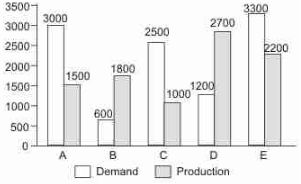What is the ratio of companies having more demand than production to those having more production than demand?

Solution:

A, C and E have more demand.
B and D have more production .
So the ratio is 3 : 2

QUESTION: 16

These questions are based on the graph which shows the Demand and Production statistics of the five companies.What is the difference between the average demand and the average production of five companies taken together ?

Solution:

Avg Demand = (3000 +600+2500+1200+3300)/5=10600/5 = 2120
Avg Production = 1840
So the Diff is 280

QUESTION: 17

A survey conducted on 5800 villagers staying in various villagers and having variuos favourite fruits .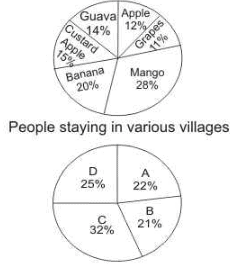Mango is the favourite fruit of 50% of the people from Village C. People having their favourite fruit as Mango from Village C form approximately what percent of the people having their faviourite fruit as mango from all the villagers together ?

Solution:
QUESTION: 18

A survey conducted on 5800 villagers staying in various villagers and having variuos favourite fruits .20% of the people from Village D have banana as their favourite fruit and 12% of the people from the same village have guava as their favourite fruit. How many people from the village like other fruits ?

Solution:

People in villave D = 25% of 5800 = 1450
∴ Required no. of people
= {100 - (20 + 12)} % of 1450
= 68% of 1450 = 986

QUESTION: 19

In the following table region-wise market share (in percentage) of Companies A, B, C for three products a, b and g is shown.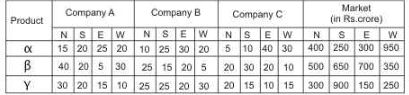Note: N= Northern region, S= Southern region, E= Eastern region, W=Western region.

What is the total sales of products α,βα,β and γγ of Companay A?

Solution:
QUESTION: 20

In the following table region-wise market share (in percentage) of Companies A, B, C for three products a, b and g is shown.Note: N= Northern region, S= Southern region, E= Eastern region, W=Western region.

If another Company D also produce αα ,β,β and γγ and has the remaining market share of the three products in all the four region, then Company D has more than 50% market share.

Solution: